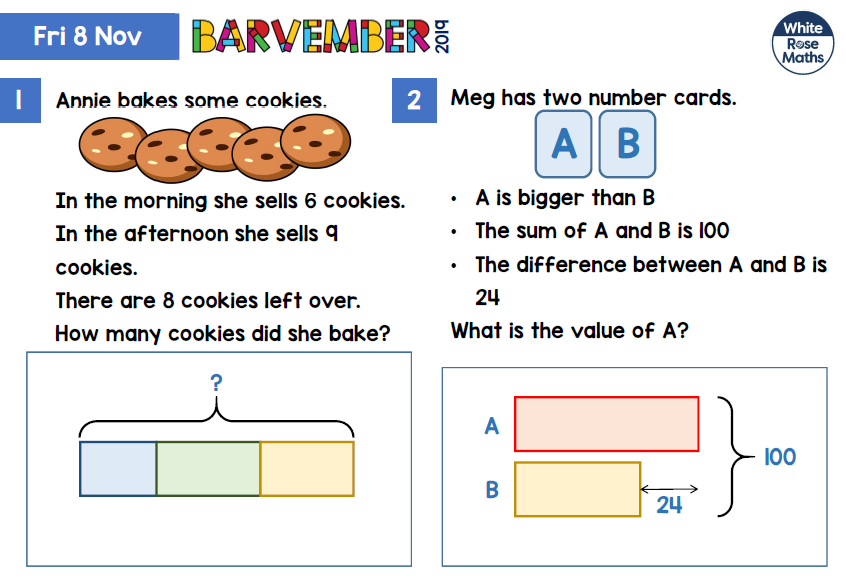### 8/11/19 Barvember challengeZara

1. Annie baked 23 biscits I know that because 6+8+9=23.
2. A+ B=100 and A= B+24.
100-2=76.
76÷2=38.
B=38
A=38+24
A= 62.

Eben

1=23
2= A is 62